Question

# What is the molar solubility of AgI in a solution of 0.020M CaI2 at 25 degrees...

What is the molar solubility of AgI in a solution of 0.020M CaI2 at 25 degrees C?
A. 2.1x10^-15 M
B. 4.3x10^-15 M
C. 8.5x10^-15 M
D. 9.2x10^-9 M
*please show work ive tried several times and keep getting the same wrong answer ?

We need at least 10 more requests to produce the answer.

0 / 10 have requested this problem solution

The more requests, the faster the answer.

All students who have requested the answer will be notified once they are available.

#### Earn Coins

Coins can be redeemed for fabulous gifts.

Similar Homework Help Questions
• ### Given that, for Agi, at 25°C, Ksp = 1.5x10-16: What is the solubility (g/mL) of Agi...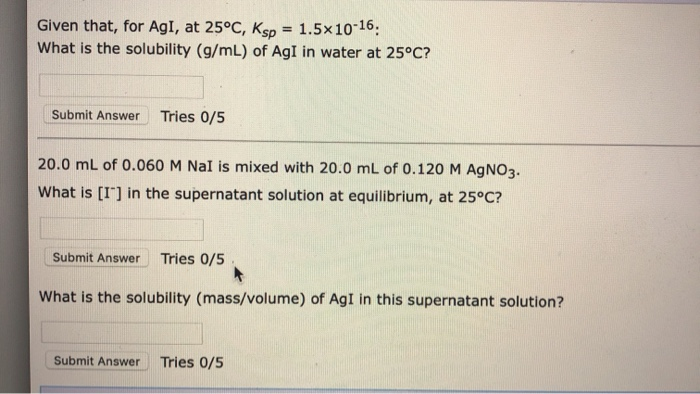Given that, for Agi, at 25°C, Ksp = 1.5x10-16: What is the solubility (g/mL) of Agi in water at 25°C? Submit Answer Tries 0/5 20.0 mL of 0.060 M Nal is mixed with 20.0 mL of 0.120 M AgNO3. What is [I"] in the supernatant solution at equilibrium, at 25°C? Submit Answer Tries 0/5 What is the solubility (mass/volume) of AgI in this supernatant solution? Submit Answer Tries 0/5

• ### What is the molar solubility of AgI in a solution which is 5.1 x 10-4 M...

What is the molar solubility of AgI in a solution which is 5.1 x 10-4 M in AgNO3? For AgI, Ksp = 8.3 x 10-17. A. 5.1 x 10-2 mol/L B. 4.2 x 10-20 mol/L C. 1.6 x 10-13 mol/L D. 1.1 x 10-5 mol/L E. 8.3 x 10-11 mol/L

• ### Calculate the molar solubility of CaF2 at 25 C in a solution that is 0.10 M...

Calculate the molar solubility of CaF2 at 25 C in a solution that is 0.10 M in Ca(NO3)2. Ksp = 3.9 E-11 for CaF2. A. 3.9 E-7 B. 1.96 E-9 C. 7.8 E-10 D. 3.9 E-11

• ### 2. Calculate the molar solubility of Ag:CrOs at 25 °C given that the Kip for the...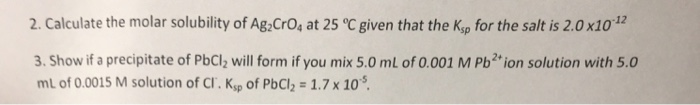2. Calculate the molar solubility of Ag:CrOs at 25 °C given that the Kip for the salt is 2.0x10 3. Show if a precipitate of PbCl2 will form if you mix 5.0 ml of 0.001 M Pb2+ion solution with 5.0 mL of 0.0015 M solution of CI. Ksp of PbCl2 1.7x 10

• ### Barium hydroxide, Ba(OH)_2 is reported to have Ksp of 1.5x10^-4 at 25 degrees Celsius. a) What...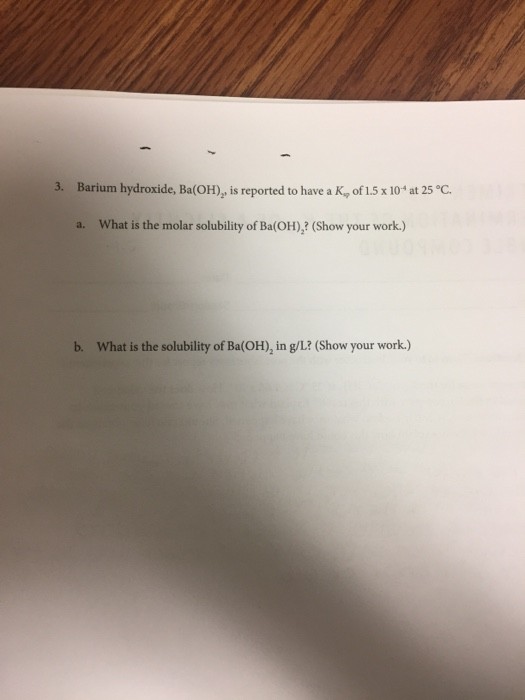Barium hydroxide, Ba(OH)_2 is reported to have Ksp of 1.5x10^-4 at 25 degrees Celsius. a) What is the molar solubility of Ba(OH)_2 b) What is the solubility of Ba(OH)_2 in g/L 3. Barium hydroxide, Ba(OH), is reported to have a K, of 1.5 x 10 at 25 C. What is the molar solubility of Ba(OH), ? (Show your work.) a. b. What is the solubility of Ba(OH), in g/L? (Show your work.)

• ### 1. The Molar Solubility of Ca(10), in Pure Water Temperature of the saturated solution of calcium...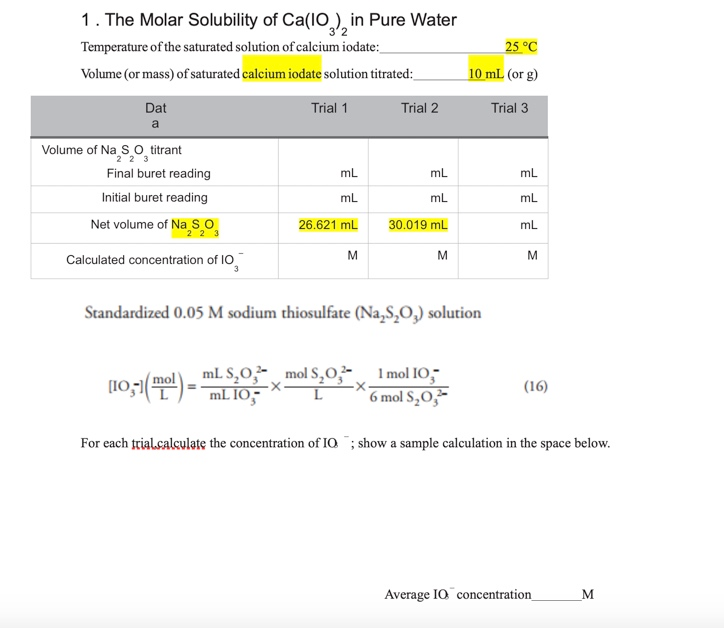1. The Molar Solubility of Ca(10), in Pure Water Temperature of the saturated solution of calcium iodate: Volume (or mass) of saturated calcium iodate solution titrated: 25 °C 10 ml (or g) Dat Trial 1 Trial 2 Trial 3 Volume of Na S o titrant Final buret reading Initial buret reading ml mL mL mL Net volume of Na So 26.621 mL 30.019 mL Calculated concentration of 10 Standardized 0.05 M sodium thiosulfate (Na,8,0,) solution (10,51(E) - TIL 10 mols.0.2...

• ### 5. (36 pts total) To determine the molar solubility and solubility product constant of magnesium carbonate,...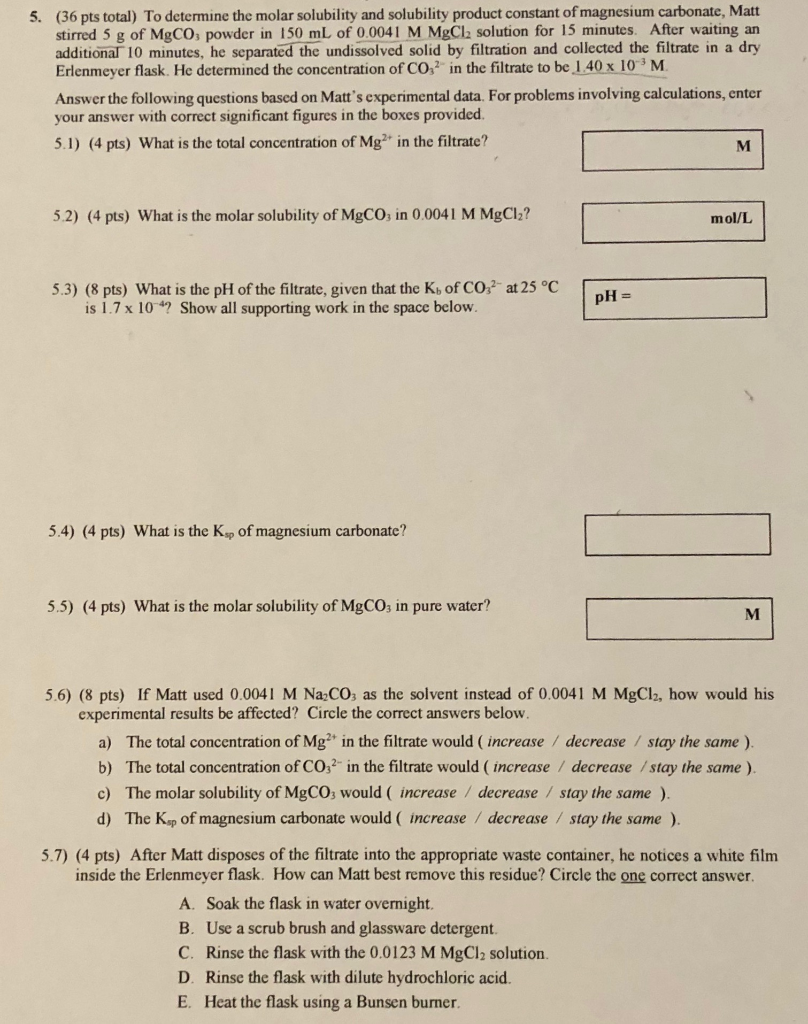5. (36 pts total) To determine the molar solubility and solubility product constant of magnesium carbonate, Matt stirred 5 g of MgCO3 powder in 150 mL of 0.0041 M MgCl2 solution for 15 minutes. After waiting an additional 10 minutes, he separated the undissolved solid by filtration and collected the filtrate in a dry Erlenmeyer flask. He determined the concentration of CO2 in the filtrate to be 1.40 x 10M Answer the following questions based on Matt's experimental data. For...

• ### 1. The Molar Solubility of Ca(10), in Pure Water Temperature of the saturated solution of calcium...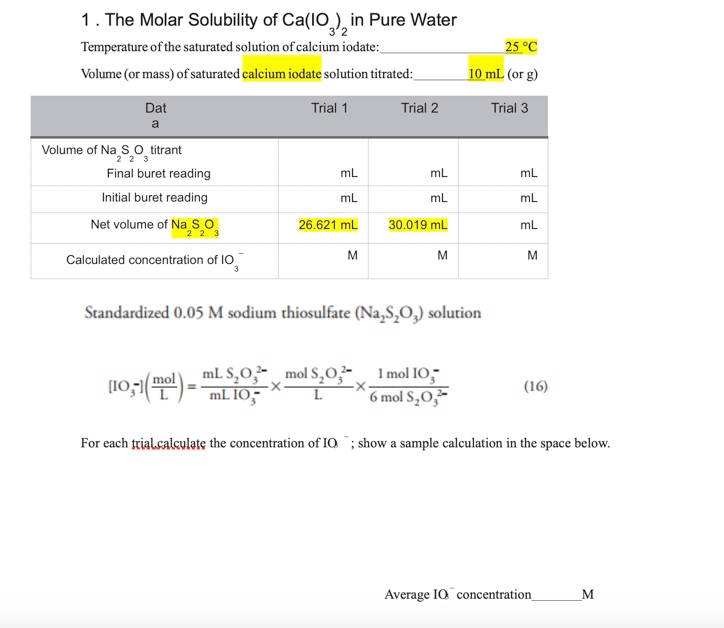1. The Molar Solubility of Ca(10), in Pure Water Temperature of the saturated solution of calcium iodate: Volume (or mass) of saturated calcium iodate solution titrated: 25 °C 10 ml (or g) Dat Trial 1 Trial 2 Trial 3 Volume of Na S o titrant Final buret reading Initial buret reading ml mL mL mL Net volume of Na So 26.621 mL 30.019 mL Calculated concentration of 10 Standardized 0.05 M sodium thiosulfate (Na,8,0,) solution (10,51(E) - TIL 10 mols.0.2...

• ### please solve and show work! Determine the molar solubility of AgBr in a solution containing 0.250...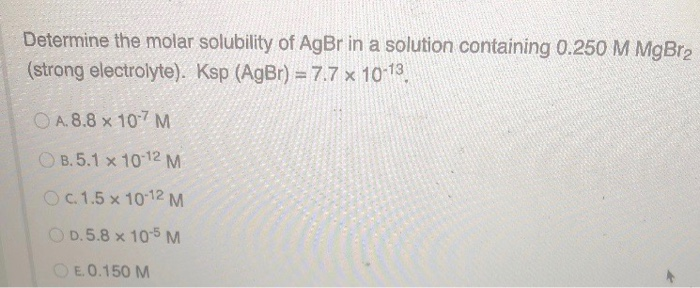please solve and show work! Determine the molar solubility of AgBr in a solution containing 0.250 M MgBr2 (strong electrolyte). Ksp (AgBr) = 7.7 x 10-13 O A.8.8 x 107 M B.5.1 x 10-12 M c. 1.5 x 10-12 M O 0.5.8 x 10-5 M E.0.150 M

• ### A25. What is the molar solubility of cobalt carbonate, CoCO3, in an aqueous solution containing 0.3...

A25. What is the molar solubility of cobalt carbonate, CoCO3, in an aqueous solution containing 0.3 M sodium carbonate at 25 °C? (Cobalt carbonate Ksp = 1.0 × 10–10) (A) 1.0 × 10–5 M (B) 5.48 × 10–6 M (C) 0.3 M (D) 3.3 × 10–10 M (E) 1.0 × 10–10 M